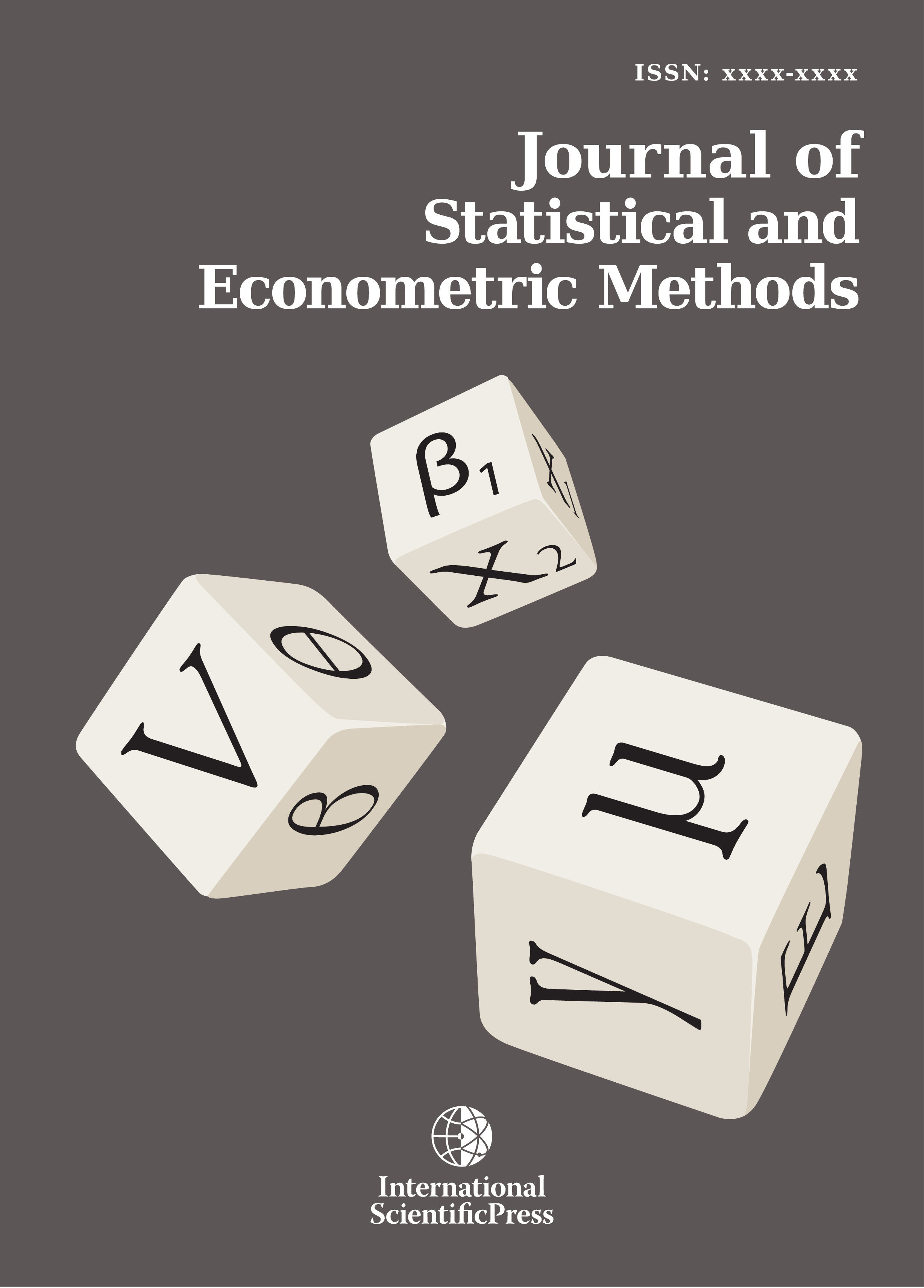# Journal of Statistical and Econometric Methods

#### Derivation of Kalman Filter Estimates Using Bayesian Theory: Application in Time Varying Beta CAPM Model

•[ Download ]
• Times downloaded: 602
• Abstract

This paper is concerned with application of Kalman recursive estimates in the the capital asset pricing (CAPM) model with time varying beta parameters. Following Kyriazis (2011), Kalman estimates are derived using a Bayesian probability theory. Rate of convergence and sensitivity analysis of estimates are derived. Through five examples, applications of presented estimates are shown. Extension to the non-normal cases and suggestion of Bayes filter is also considered. Comparisons with method of moment estimates are given.ISSN: 2241-0376 (Online)
2241-0384 (Print)